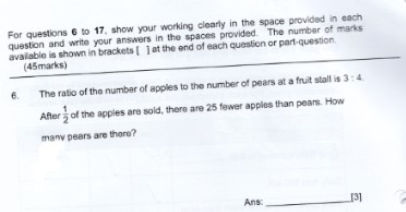# QuestionThe ratio of the number of apples to the number of pears at a fruit stall is 3:4.After 1/2 of the apples are sold,there are 25 fewer apples than pears.How many pears are there?

How to tackle this question?

Apples                    Pears

Initially                                         3u                            4u

1/2 apples sold                         -(3/2)u

Final                                        3u-(3/2)u                     4u

Apples are is now 25 fewer than pears => to equal pears now, +25 to apple

3u-(3/2)u + 25 = 4u      (x 2 for both sides)

6u – 3u + 50 = 8u

5u = 50 => u = 10

Hence number of pears = 4u = 4 x 10 = 40

0 Replies 1 Like

If number of apples is 6U initially , then

Apples                                                 Pears

Initially                                    6U                                                       8U

Finally                                     3U                                                       8U

Given 8U-3U=25

U=5

So there are 5*8=40 pears

The ratio of apple is 3 at first , but it will be half later, so it is better to use 6 so that after halving you still have an integer to work with.

0 Replies 1 Like